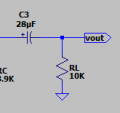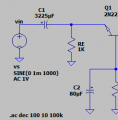# Common-Base Voltage Amplifier - Capacitors

#### aac044210

Joined Nov 19, 2019
178
Since I have never worked with a common-base amplifier before,
I just wanted to confirm that the coupling capacitors and bypass capacitor
values have been correctly calculated for the attached voltage amplifier.

f1 = 20Hz, Zin = 24.2Ω, Zout = 2.8KΩ, RB2 = 1KΩ

C1 = 1 / 2 * π * 20Hz * Zin * 0.1 = 3,288μF

C2 = 1 / 2 * π * 20Hz * RB2 * 0.1 = 80μF

C3 = 1 / 2 * π * 20Hz * Zout * 0.1 = 28μF

Thanks

#### Attachments

• 3 KB Views: 13

#### ronsimpson

Joined Oct 7, 2019
2,261
C2 = 1 / 2 * π * 20Hz * RB2 * 0.1 = 80μF
I think "RB2" should be "RB2//RB1" parallel of the two resistors.
C3 = 1 / 2 * π * 20Hz * Zout * 0.1 = 28μF
Change "Zout" to "RL". The capacitor is loaded by RL=10k.

#### aac044210

Joined Nov 19, 2019
178
I think "RB2" should be "RB2//RB1" parallel of the two resistors.

Change "Zout" to "RL". The capacitor is loaded by RL=10k.
Thanks Ron.
I am still concerned about C1, wondering if the resistance should be Zin or RE.
Zin results in such a large capacitor.

Thanks Ron!

Last edited:

#### ronsimpson

Joined Oct 7, 2019
2,261
I did a frequency response at the Emitter and it looks right.
The current through C1 goes mostly into the Emitter (Zin=24) and a little goes into RE=1k. So really it is Zin//RE. In the real world RE has little effect and I did not include it before. But in school I think you should say Zin parallel with RE.

#### Jony130

Joined Feb 17, 2009
5,405
I am still concerned about C1, wondering if the resistance should be Zin or RE.
Zin = r'e||RE ≈ r'e if RE >> r'e

The impedance seen by C3 is (Rc + RL).

#### aac044210

Joined Nov 19, 2019
178
Zin = r'e||RE ≈ r'e if RE >> r'e

The impedance seen by C3 is (Rc + RL).
So these are correct?
C1 = 1 / 2 * π * 20Hz * Zin * 0.1 = 3,183μF

C2 = 1 / 2 * π * 20Hz * RB * 0.1 = 80μF

C3 = 1 / 2 * π * 20Hz * (RC + RL) * 0.1 = 6μF

where Zin = 25Ω, RB = 822Ω, and RC + RL = 13.9KΩ

Last edited:

#### ronsimpson

Joined Oct 7, 2019
2,261
C3 = 1 / 2 * π * 20Hz * (RC + RL) * 0.1 = 6μF
C3 is only this circuit, no more. No RC.C2 = 1 / 2 * π * 20Hz * RB * 0.1 = 80μF
C2 sees RB2 to ground and it sees RB1 to 10V which is a short to ground. In the AC model RB2 is parallel with RB1.C1 = 1 / 2 * π * 20Hz * Zin * 0.1 = 3,183μF
C1= the impedance of the emitter and it also see RE. Zin//RE.#### Jony130

Joined Feb 17, 2009
5,405
So these are correct?
C1 = 1 / 2 * π * 20Hz * Zin * 0.1 = 3,183μF

C2 = 1 / 2 * π * 20Hz * RB * 0.1 = 80μF

C3 = 1 / 2 * π * 20Hz * (RC + RL) * 0.1 = 6μF

where Zin = 25Ω, RB = 822Ω, and RC + RL = 13.9KΩ
Looks good. And C1 is large because you set your 3dB corner frequency at 2Hz and Zin is very low resistance.

And in real life pick the capacitor values from this series:

10, 22, 33, 47, 51, 68

So we have

C1 = 3300μF
C2 = 100μF
C3 = 6.8μF or 10μF (better).

Because those values are easy to buy in reality.

C3 is only this circuit, no more. No RC.
How can Rc do not have any influence on C3 time constant?